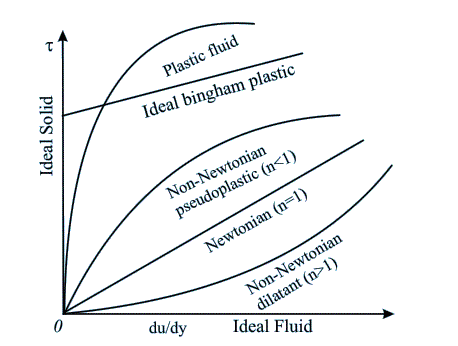# Fluids & Types of Fluid

## What is a fluid?

Matter around us exists in three phases (excluding plasma)
1.       Solid
2.       Liquid
3.       Gas
From the above three phases liquid and gas are combinedly known as fluids.
The main difference between fluids and solid lies in their ability to resist shear stresses.
When a constant shear force is applied, a solid eventually stops deforming, whereas a fluid never stops deforming and approaches a constant rate of strain
(ref. fluid mechanics by Cengel & Cimbala)

## Types of fluids

On the basis of shear and velocity gradient relationship, fluids can broadly be classified into following five types.Types of fluids

1.       Ideal fluid
Ideal fluid is incompressible and has no viscosity. It is an imaginary fluid and does not exists in reality.
2.       Real fluid
Real fluid possesses viscosity. In practice all fluids are real.
3.       Newtonian fluid
A real fluid which obeys Newton’s law of viscosity is known as Newtonian fluid.
Note: According to Newton’s law of viscosity shear stress is directly proportional to strain rate.
4.       Non-Newtonian fluids
A real fluid which does not obeys Newton’s law of viscosity is known as Non-Newtonian fluid.
5.       Ideal plastic fluid
A fluid, in which shear stress is more than the yield value and shear stress to proportional to the rate of shear strain, is known as real plastic fluid.

## Properties of fluids

There are some basis properties associated with fluids which help us to understand them in a better way.
1.       Viscosity
It is the ratio of applied shear stress to the obtained shear strain rate in fluids. Viscosity offers resistance to the movement of fluid layers.
2.       Pressure
It is the term used in fluids which is analogues to the term stress used in solids. Both are the ratio of applied force and area of surface (on which the force is applied)
3.       Specific gravity
Specific gravity of fluids is defined as the ratio of density of a particular fluid to the density of standard fluid (generally water).

## Examples of fluids

Water
Air
Blood
Mercury
Honey
Gasoline
Any other gas or liquid